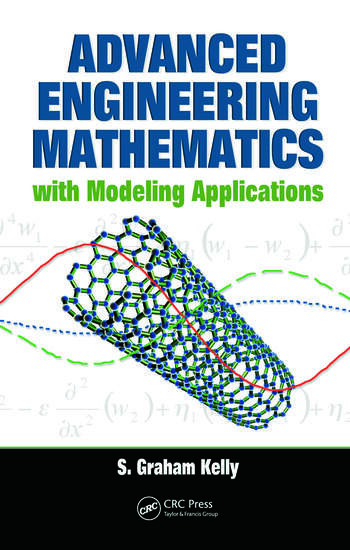Advanced Engineering Mathematics with Modeling Applications

1st Edition

S. Graham Kelly

CRC Press
Published December 5, 2008
Textbook - 522 Pages - 159 B/W Illustrations
ISBN 9780849395338 - CAT# 9533

For Instructors Request Inspection Copy

was \$145.00

USD\$116.00

SAVE ~\$29.00

FREE Standard Shipping!

Preview

Summary

Engineers require a solid knowledge of the relationship between engineering applications and underlying mathematical theory. However, most books do not present sufficient theory, or they do not fully explain its importance and relevance in understanding those applications.

Advanced Engineering Mathematics with Modeling Applications employs a balanced approach to address this informational void, providing a solid comprehension of mathematical theory that will enhance understanding of applications – and vice versa. With a focus on modeling, this book illustrates why mathematical methods work, when they apply, and what their limitations are. Designed specifically for use in graduate-level courses, this book:

• Emphasizes mathematical modeling, dimensional analysis, scaling, and their application to macroscale and nanoscale problems
• Explores eigenvalue problems for discrete and continuous systems and many applications
• Develops and applies approximate methods, such as Rayleigh-Ritz and finite element methods
• Presents applications that use contemporary research in areas such as nanotechnology

Apply the Same Theory to Vastly Different Physical Problems
Presenting mathematical theory at an understandable level, this text explores topics from real and functional analysis, such as vector spaces, inner products, norms, and linear operators, to formulate mathematical models of engineering problems for both discrete and continuous systems. The author presents theorems and proofs, but without the full detail found in mathematical books, so that development of the theory does not obscure its application to engineering problems. He applies principles and theorems of linear algebra to derive solutions, including proofs of theorems when they are instructive. Tying mathematical theory to applications, this book provides engineering students with a strong foundation in mathematical terminology and methods.

Instructors

We provide complimentary e-inspection copies of primary textbooks to instructors considering our books for course adoption.